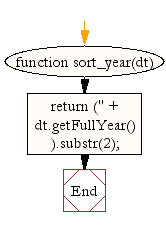# JavaScript: Get a two digit representation of a year

## JavaScript Datetime: Exercise-29 with Solution

Write a JavaScript function to get a two digit representation of a year.

Test Data:
dt = new Date();
console.log(sort_year(dt));
18
dt = new Date(1989, 10, 1);
console.log(sort_year(dt));
89

Sample Solution:

HTML Code:

``````<!DOCTYPE html>
<html>
<head>
<meta charset="utf-8">
<title>JavaScript function to get a two digit representation of a year</title>
</head>
<body>

</body>
</html>
```
```

JavaScript Code:

``````function sort_year(dt)
{
return ('' + dt.getFullYear()).substr(2);
}

dt = new Date();
console.log(sort_year(dt));

dt = new Date(1989, 10, 1);
console.log(sort_year(dt));
```
```

Sample Output:

```18
89
```

Flowchart:Live Demo:

See the Pen JavaScript - Get a two digit representation of a year-date-ex-29 by w3resource (@w3resource) on CodePen.

Improve this sample solution and post your code through Disqus

What is the difficulty level of this exercise?

﻿

## JavaScript: Tips of the Day

Convert tabs to spaces, where each tab corresponds to count spaces

Example:

```const tips_expandTabs = (str, count) => str.replace(/\t/g, ' '.repeat(count));
console.log(tips_expandTabs('\t\tw3resource', 4));
```

Output:

```"        w3resource"
```